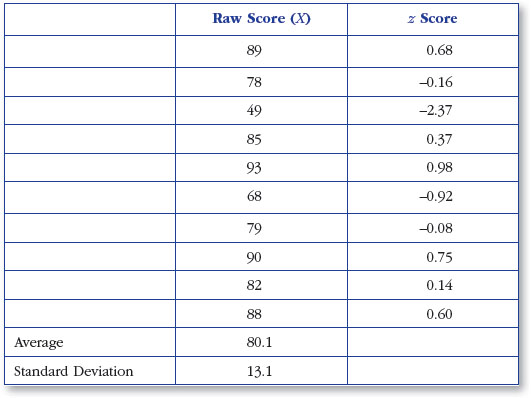In an increasingly data-driven world, it is more important than ever for students as well as professionals to better understand basic statistical concepts. 100 Questions (and Answers) About Statistics addresses the essential questions that students ask about statistics in a concise and accessible way. It is perfect for instructors, students, and practitioners as a supplement to more comprehensive materials, or as a desk reference with quick answers to the most frequently asked questions.

# How Can Excel Be Used to Compute z Scores?

### How Can Excel Be Used to Compute z Scores?

Using Excel to compute z scores is a simple procedure that can be done by following these steps. After completing the four steps below, your worksheet should look as it appears in Figure 71.1.

### Figure 71.1 Using Excel to compute z scores• List all the raw scores in a column in a new worksheet. Label the column “Raw Score (X),” as you see in Figure 71.1.
• Using the =AVERAGE function, compute the mean and place it at the bottom of the column.
• Using the =STDEV.S function, compute the standard deviation and place it below the mean. You can see steps 2 and 3 completed in Figure 71.1.
• In cell C2, place the following formula . . .

$z=\phantom{\rule{0.5em}{0ex}}\left(\text{B}2\phantom{\rule{0.5em}{0ex}}–\phantom{\rule{0.5em}{0ex}}\text{B}12\right)/\text{B}13$

and then copy ...

•••